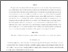# Power Allocation For Outage Minimization in State Estimation Over Fading Channels

Leong, Alex S. and Dey, Subhrakanti and Nair, Girish N. and Sharma, Priyank (2011) Power Allocation For Outage Minimization in State Estimation Over Fading Channels. IEEE Transactions on Signal Processing, 59 (7). pp. 3382-3397. ISSN 1053-587XPreviewmore...Add this article to your Mendeley library

## Abstract

This paper studies the outage probability minimization problem for state estimation of linear dynamical systems using multiple sensors, where an estimation outage is deﬁned as an event when the state estimation error exceeds a pre-determined threshold. The sensors amplify-and-forward their measurements (using uncoded analog transmission) to a remote fusion center over wireless fading channels. For stable systems, the resulting inﬁnite horizon problem can be formulated as a constrained average cost Markov decision process (MDP) control problem. A suboptimal power allocation that is less computationally intensive is proposed, and numerical results demonstrate very close performance to the power allocation obtained from the solution of the MDP based average cost optimality equation. Motivated by practical considerations, assuming that sensors can transmit with only a ﬁnite number of power levels, optimization of the values of these levels is also considered using a stochastic approximation technique. In the case of unstable systems, a ﬁnite horizon formulation of the estimation outage minization problem is presented and solved. An extension to the problem of minimization of the expected error covariance is also studied.

Item Type: Article Power Allocation; Outage; Minimization; State Estimation; Fading Channels; Faculty of Science and Engineering > Electronic Engineering 12711 https://doi.org/10.1109/TSP.2011.2135350 Subhrakanti Dey 06 Apr 2020 11:21 IEEE Transactions on Signal Processing Institute of Electrical and Electronics Engineers YesItem control page